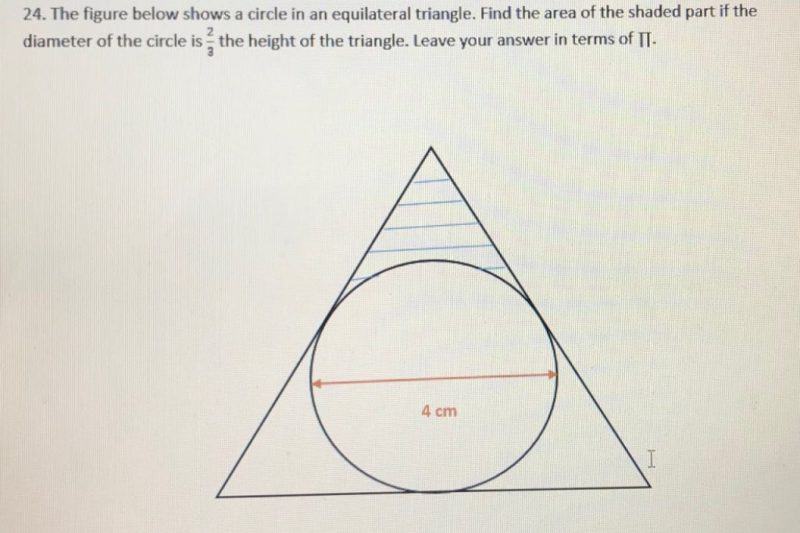# Question4 = (2/3) x height of the triangle
height of triangle = 6
Let the length of one side of the equilateral triangle be 2a.
Using Pythagoras ‘ theorem,
a^2 + 2^2 = 4^2
a = sqrt 12
2a = 2(sqrt 12)
area of triangle = (1/2) x 2(sqrt 12) x 6 = 6(sqrt 12)
area of circle = pi x 2 x 2 = 4pi
area of shaded part = [6(sqrt 12) – 4pi]/3 = 2(sqrt 12) – (4pi/3)

Ans : 2(sqrt 12) – (4pi/3) sq cm.

0 Replies 0 Likes

If you don’t want to use Pythagoras’ Theorem, then you will need to use similar triangles to find the side of the equilateral triangle

0 Replies 0 Likes

Assuming the question is correct,

Height of triangle = 3/2 of diameter of circle = 6 cm

Let the length of the equilateral triangle be 2x cm.

Using Pythagaros’ Theorem,

4x2 = x2 + 36

3x2 = 36

x = 2 √ 3

Length of equilateral triangle = 4√ 3  cm

Area of equilateral triangle = 1/2 x 4√ 3 x 6 = 12√ 3  cm2

Area of circle = 4π

Shaded area = 1/3 (12√ 3 – 4π) cm2 = 4√ 3 – 4/3π  cm2

0 Replies 0 Likes

Is it really height of the triangle?? If it’s the side, you can get it without square root.

Height : 2*sqrt(12) – 3/4Pi

If it’s a Side : 6 – 3/4Pi

0 Replies 0 Likes